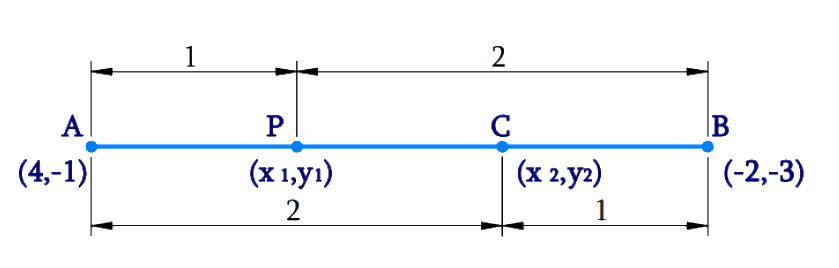# Ex.7.2 Q2 Coordinate Geometry Solution - NCERT Maths Class 10

Go back to  'Ex.7.2'

## Question

Find the coordinates of the points of trisection of the line segment joining $$(4, -1)$$ and $$(-2, -3)$$.

## Text Solution

Reasoning:

The coordinates of the point $$P(x, y)$$ which divides the line segment joining the points $$A(x1, y1)$$ and $$B(x2, y2)$$, internally, in the ratio $$\rm m1 : m2$$ is given by the Section Formula.

What is Known?

The $$x$$ and $$y$$ co-ordinates of the line segment joining $$(4, -1)$$ and $$(-2, -3)$$.

What is Unknown?

The coordinates of the points of trisection of the line segment joining $$(4, -1)$$ and $$(-2, -3)$$.

Steps:Given,

• Let line segment joining the points be $$A(4, -1)$$ and $$B(-2, -3)$$.
• Let $$P \;(x1, y1)$$ and $$Q\; (x2, y2)$$ be the points of trisection of the line segment joining the given points i.e., $$AP = PQ = QB$$

By Section formula

\begin{align}{\text{P(x,y)}} = \left[ {\frac{{{\text{m}}{{\text{x}}_2} + {\text{n}}{{\text{x}}_1}}}{{{\text{m}} + {\text{n}}}},\frac{{{\text{m}}{{\text{y}}_2} + {\text{n}}{{\text{y}}_1}}}{{{\text{m}} + {\text{n}}}}} \right] & & ...\,{\text{Equation}}\,{\text{(1)}}\end{align}

Therefore, by observation point $$P$$ divides $$AB$$ internally in the ratio $$1:2$$.

• Hence $$\rm m: n = 1:2$$

By substituting the values in the Equation (1)

\begin{align}{{\text{x}}_1} &= \frac{{1 \times ( - 2) + 2 \times 4}}{{1 + 2}}, \qquad {{\text{y}}_1} = \frac{{1 \times ( - 3) + 2 \times ( - 1)}}{{1 + 2}}\\{{\text{x}}_1} &= \frac{{ - 2 + 8}}{3} = \frac{6}{3} = 2, \qquad {{\text{y}}_1} = \frac{{ - 3 - 2}}{3} = \frac{{ - 5}}{3}\end{align}

Therefore, \begin{align}{\text{P}}\left( {{{\text{x}}_1},{{\text{y}}_1}} \right) = \left( {2,\frac{{ - 5}}{3}} \right)\end{align}

Therefore, by observation point $$Q$$ divides $$AB$$ internally in the ratio $$2:1$$.

• Hence $$\rm m:n = 2:1$$

By substituting the values in the Equation (1)

\begin{align}{{\text{x}}_2} &= \frac{{2 \times ( - 2) + 1 \times 4}}{{2 + 1}}, \qquad{{\text{y}}_2} = \frac{{2{\text{x}}( - 3) + 1 \times ( - 1)}}{{2 + 1}}\\{{\text{x}}_2} &= \frac{{ - 4 + 4}}{3} = 0, \qquad \qquad\;\; {{\text{y}}_2} = \frac{{ - 6 - 1}}{3} = \frac{{ - 7}}{3}\end{align}

Therefore, \begin{align}{\text{Q}}\;\left( {{{\text{x}}_2},{{\text{y}}_2}} \right) = \left( {0, - \frac{7}{3}} \right)\end{align}

Hence the points of trisection are\begin{align}P\left( {{{\text{x}}_1},{{\text{y}}_1}} \right) = \left( {2,\frac{{ - 5}}{3}} \right) \text{and}\;Q\left( {{{\text{x}}_2},{{\text{y}}_2}} \right) = \left( {0, - \frac{7}{3}} \right)\end{align}

Learn from the best math teachers and top your exams

• Live one on one classroom and doubt clearing
• Practice worksheets in and after class for conceptual clarity
• Personalized curriculum to keep up with school